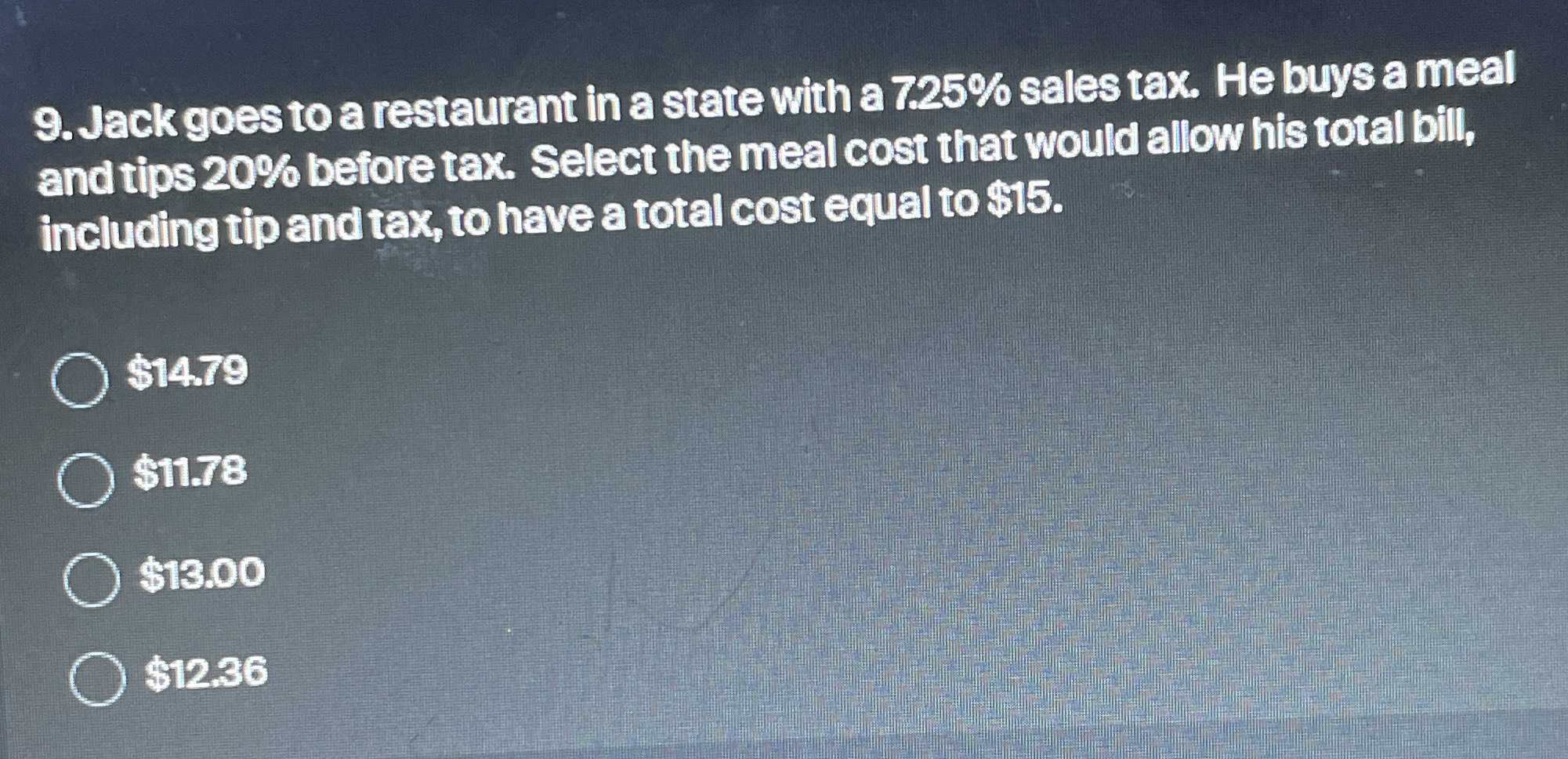### Still have math questions?

Arithmetic
Question9. Jack goes to a restaurant in a state with a $$7.25 \%$$ sales tax. He buys a meal and tips $$20 \%$$ before tax. Select the meal cost that would allow his total bill, including tip and tax, to have a total cost equal to $$\ 15$$ .

$$\ 14.79$$

$$\ 11.78$$

$$\ 13.00$$

$$\ 12.36$$